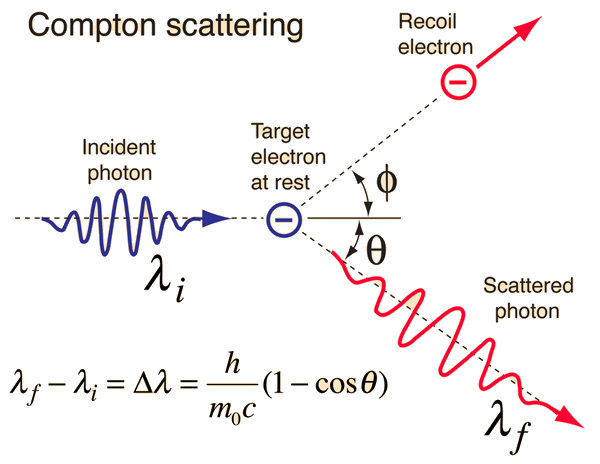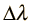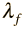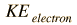The scattering of photons from charged particles is called Compton scattering after Arthur Compton who was the first to measure photon-electron scattering in 1922. When the incoming photon gives part of its energy to the electron, then the scattered photon has lower energy and according to the Planck relationship has lower frequency and longer wavelength. The wavelength change in such scattering depends only upon the angle of scattering for a given target particle. The constant in the Compton formula above can be writtenand is called the Compton wavelength for the electron. The formula presumes that the scattering occurs in the rest frame of the electron.

For an incoming wavelength= nm = x 10^ m
 scattered at angle θ=degrees

the change in wavelength is= nm = x 10^ m

and the final wavelength is= nm = x 10^ m

Expressed in terms of photon energies, the incoming photon energy is= x 10^ eV = x 10^ joules
 =eV = keV = MeV
and the scattered photon energy is= x 10^ eV = x 10^ joules
 =eV = keV = MeV

This implies that the energy transferred to the electron is= x 10^ eV = x 10^ joules
 =eV = keV = MeV .

This exploration is designed to accept values for the input wavelength or energy and the angle of scattering. Unspecified parameters will default to the case of molybdenum K-alpha xrays scattering at 90 degrees, one of Compton's historical results. Any of these input values can be changed.

 Compton Scattering Data Derivation of equation
 Significance of Compton scattering
 Extreme case of highly relativistic photon
Index

Great experiments of physics

Reference:
Compton

 HyperPhysics***** Quantum Physics R Nave
Go Back